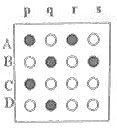## Monday, April 30, 2007

### IIT-JEE 2007 Matrix-Match Type Question on Modern Physics

Section IV of Part I Physics question paper of IIT-JEE 2007 contained 3 Matrix-Match Type questions. Each question contained statements given in two columns. Statements A,B,C,D in Column I had to be matched with statements p,q,r,s in Column II. The answers to the questions had to be appropriately bubbled as shown at the end of this post.

Here is the question involving topics in modern physics:

Some laws / processes are given in Column I. Match these with the physical phenomena given in Column II and indicate your answer by darkening appropriate bubbles in the 4 ×4 matrix in the ORS

Column I Column II

(A) Transition between (p) Characteristic X- rays

two atomic energy levels
(B) Electron emission from (q) Photoelectric effect

a material

(C) Mosley’s law (r) Hydrogen spectrum

(D) Change of photon (s) β-decay

energyCharacteristic X-rays and hydrogen spectrum are produced by electron transition between two energy levels in an atom. So, (A) is to be matched with (p) and (r).

In photo electric effect and β-decay, electrons are emitted from a material. So, (B) is to be matched with (q) and (s).

Mosley’s law [√f α Z ] relates the frequency ‘f’ of a particular characteristic X-ray (e.g., Kα ) to the atomic number Z of the target in the X-ray tube. So, (C) is to be matched with (p).

In photoelectric effect, the energy of the incident photon is used in dislodging electrons from a photo sensitive surface. So, (D) is to be matched with (q).

The appropriate bubbles darkened are shown in figure.